# Test: Circles- 2

## 6 Questions MCQ Test Practice Questions for GMAT | Test: Circles- 2

Description
Attempt Test: Circles- 2 | 6 questions in 10 minutes | Mock test for GMAT preparation | Free important questions MCQ to study Practice Questions for GMAT for GMAT Exam | Download free PDF with solutions
QUESTION: 1

### If 1/a2 + a2 represents the diameter of circle O and 1/a + a = 3, which of the following best approximates the circumference of circle O?

Solution:

Let’s first consider the relationship between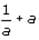and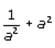. If we square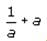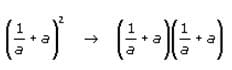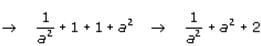We can use this information to manipulate the equation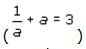provided in the question. If we square both sides of the given equation, we arrive at the following: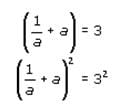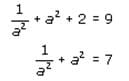The question asks for the approximate circumference of a circle with a diameter of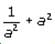which we have determined is equal to 7.

Circumference = diameter x π = 7π≈ 22

QUESTION: 2

### Two circular road signs are to be painted yellow. If the radius of the larger sign is twice that of the smaller sign, how many times more paint is needed to paint the larger sign (assuming that a given amount of paint covers the same area on both signs)?

Solution:

If we know the ratio of the radii of two circles, we know the ratio of their areas. Area is based on the square of the radius [A = π (radius)2]. If the ratio of the radii of two circles is 2:1, the ratio of their areas is 22: 12 or 4:1.

In this question, the radius of the larger circular sign is twice that of the smaller circular sign;
In this question, the radius of the larger circular sign is twice that of the smaller circular sign; therefore the larger sign's area is four times that of the smaller sign and would require 4 times as much paint.

QUESTION: 3

### In the diagram, points A, B, and C are on the diameter of the circle with center B. Additionally, all arcs pictured are semicircles. Suppose angle YXA = 105 degrees. What is the ratio of the area of the shaded region above the line YB to the area of the shaded region below the line YB? (Note: Diagram is not drawn to scale and angles drawn are not accurate.)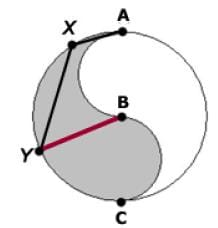Solution:

Since the question asks for a ratio, it will be simplest to define the radius of the circle as 1.
Inscribed angle AXY (given as 105¨ intercepts arc ACY. By definition, the measure of an inscribed angle is equal to half the measure of its intercepted arc. Thus arc ACY = 2 x 105 = 210

We are given that points A, B and C are all on the diameter of the circle. Using this information, we can think of arc ACY in two chunks: arc AC plus arc CY. Since arc AC defines a semicircle, it is equal to 1800. Therefore arc CY = 2100 – 1800 = 300

We can now use a proportion to determine the area of sector CBY. Since arc CY represents 30/360 or 1/12 the measure of the entire circle, the area of sector CBY = 1/12 the area of the entire circle. Given that we have defined the circle as having a radius of 1, the area of sector CBY = (1/12) πr2 = (1/12)π(1)2 = π /12.

Next, we must determine the area of the small gray semi-circle with diameter BC. Since BC = 1, the radius of this semi-circle is .5. The area of this semi-circle is 1/2π r2 = (1/2)π (.5)2 = π /8.

Thus, the area of the shaded region below the red line is π /12 + π /8 = 5 π/24.

Now we must determine the area of the shaded region above the red line. Using the same logic as above, angle YBA is 1800 – 300 = 1500. We can now use a proportion to determine the area of sector YBA.
Since arc YA represents 150/360 or 5/12 the measure of the entire circle, the area of sector YBA = 5/12 the area of the entire circle. Given that we have defined the circle as having a radius of 1, the area of sector YBA = (5/12)r2 = (5/12)π(1)2 = 5π/12.
We must now determine the area of the small white semi-circle with diameter AB. Since AB = 1, the radius of this semi-circle is .5. The area of this semi-circle is 1/2πr2 = (1/2)π(.5)2= π/8
Thus, the area of the shaded region above the red line is 5π/12 - π/8 = 7π/24
Finally, then, the ratio of the two areas is (7π/24)/(5π/24) = 7/5. The correct answer is D.

QUESTION: 4

Two circles share a center at point C, as shown. Segment AC is broken up into two shorter segments, AB and BC, with dimensions shown. What is the ratio of the area of the large circle to the area of the small circle?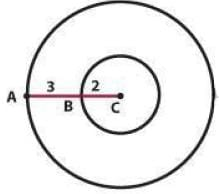Solution:

The radius of the small circle is 2.  Calculating the area gives:
Area = πr2
Area small circle =π(2)2 = 4π
The radius of the large circle is 5.  Calculating the area gives:
Area = πr2
Area large circle  = π(5)2 = 25π
So, the ratio of the area of the large circle to the area of the small circle is:
25π/4π = 25/4

QUESTION: 5

The length of minor arc AB is twice the length of minor arc BC and the length of minor arc AC is three times the length of minor arc AB. What is the measure of angle BCA?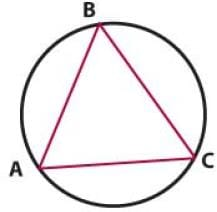Solution:

According to the question, the ratio of the lengths of arc AC to arc AB to arc BC is 6: 2: 1. An inscribed angle cuts off an arc that is twice its measure in degrees. For example, if angle ACB is 30°, minor arc AB is 600 (or 60/360 = 1/6 of the circumference of the circle).
The angles of triangle ABC are all inscribed angles of the circle, so we can deduce the ratio of the angles of triangle ABC from the ratio of the lengths of minor arcs AC, AB and BC. The ratio of the angles will be the same as the ratios of the arcs.
The ratio of angle ABC to angle BCA to angle BAC is also 6: 2: 1.  Using the unknown multiplier strategy, whereby a variable can be multiplied by each segment of a ratio to define the actual values described by that ratio, we might say that the interior angles equal 6x: 2x: x.
The sum of the angles in a triangle is 180, so x + 2x + 6x = 180.
9x = 180
x = 20
Angle BCA = 2x or 40

QUESTION: 6

In the figure, circle O has center O, diameter AB and a radius of 5. Line CD is parallel to the diameter. What is the perimeter of the shaded region?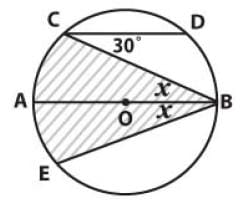Solution:

Given that line CD is parallel to the diameter, we know that angle DCB and angle CBA are equal. Thus x = 30°.

First, let's calculate the length of arc CAE. Since arc CAE corresponds to an inscribed angle of 60° (2x = 2×30° = 60°), it must correspond to a central angle of 120° which is 1/3 of the 360° of the circle. Thus we can take 1/3 of the circumference to give us the arc length CAE. The circumference is given as 2πr, where r is the radius. Thus the circumference equals 10π and arc length CAE equals (10/3)π.

Now we need to calculate the length of CB and BE. Since they have the same angle measure, these lengths are equal so we can just find one length and double it. Let us find the length of CB. If we draw a line from A to C we have a right traingle because any inscribed triangle that includes the diameter is a right triangle. Also, we know that x = 30° so we have a 30-60-90 triangle. The proportions of the length of the sides of a 30-60-90 triangle are 1- √3 - 2 for the side opposite each respective angle. We know the hypotenuse is the diameter which is 2*r = 10. So length AC must equal 5 and length CB must equal 5√3
Putting this all together gives us (10/3)π+2 x 5√3  = (10/3)π + 10√3Use Code STAYHOME200 and get INR 200 additional OFF Use Coupon Code